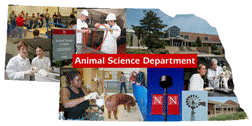Animal Science DepartmentFaculty Papers and Publications in Animal Science

Date of this Version

June 1993

Published in Journal of Dairy Science Vol. 76, No. 10. 1993.

Abstract

A methodology was developed for estimating the parameters involved in a first-order autoregressive process; these parameters comprise a variance component associated with the random effect, a correlation coefficient, p, and a residual variance. These parameters were estimated using REML with an expectation maximization algorithm. For two single trait analyses (milk and fat production being the dependent variable), the example chosen for the analyses was year-month -- treated as random and following a first-order autoregressive process within fixed herd. Initially, estimates failed to converge, possibly because of a time trend in the data, which was not accounted for by the model. After the random effect that follows the first-order autoregressive process was redefined as month within fixed herd-year, the parameters converged, and p was estimated as .8 for milk and fat yield. Results suggest that the estimation procedures may be useful for situations when a first-order autoregressive process seems appropriate.

COinS